Technical Information Site of Power Supply Design

2017.12.21 Transfer Function

# Example of Derivation for a Step-Down Converter

DC/DC Converters: Sharing of Transfer Functions among Control Systems

This is the second section of the new chapter on "Transfer Functions for Converters". We shall be deriving specific transfer functions. This time, we present an example of derivation for a step-down converter. Please read this section referring to the previous chapter as appropriate.

Example of Derivation of Transfer Functions for a Step-Down Converter

As explained previously, the transfer functions to be derived are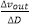and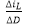. In deriving the transfer functions for the step-down converter, two steps are required.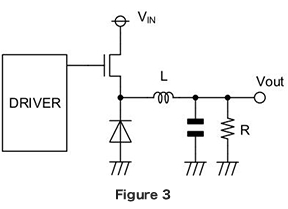●Step 1: Consider the stable states of the system

This is the procedure explained in the previous chapter. Figure 3 is the basic circuit of a diode-rectified step-down converter. The stable states of the system are the two states indicated below, each described by an equation and in a diagram. The equations are derived from equations 4-28 to 4-31, derived in “ Switching Transfer Functions: State-Space Averaging Method – From Another Viewpoint”.

① The coil current does not change over one period
② The capacitor charge amount does not change over one period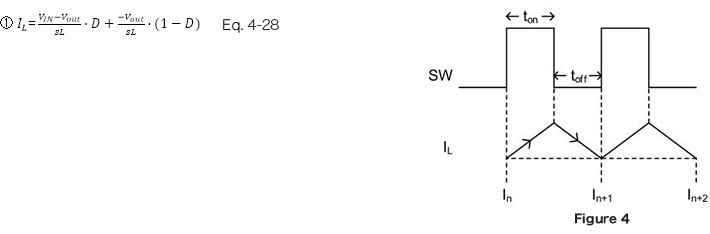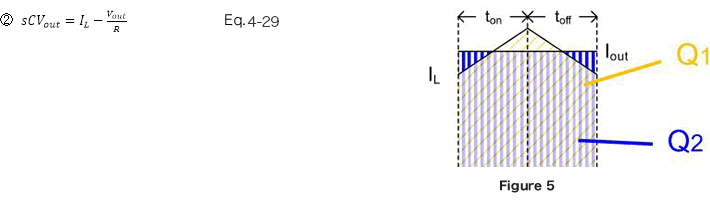●Step 2: Determine change amounts for an external disturbance, and describe the transfer functions

A calculation example is shown below.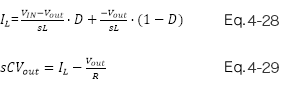Upon substituting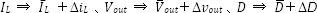in equations 4-28 and 4-29, the following is obtained.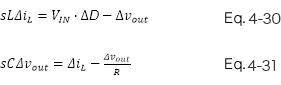Taking equations 4-30 and 4-31 to be a system of simultaneous equations and solving for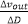and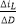, we obtain the following.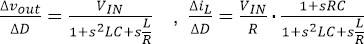From these two equations, we can derive the transfer functions for both the current mode and the voltage mode of a step-down converter.

## Key Points

・Δvout/ΔD、ΔiL/ΔD are derived for each converter.

・Transfer functions are derived in two steps, first considering the stable states of the system, and then determining change amounts for disturbances.

・In this section, the transfer functions for a step-down converter in the current and voltage modes were derived.